# MOS管栅极电阻的功耗该如何计算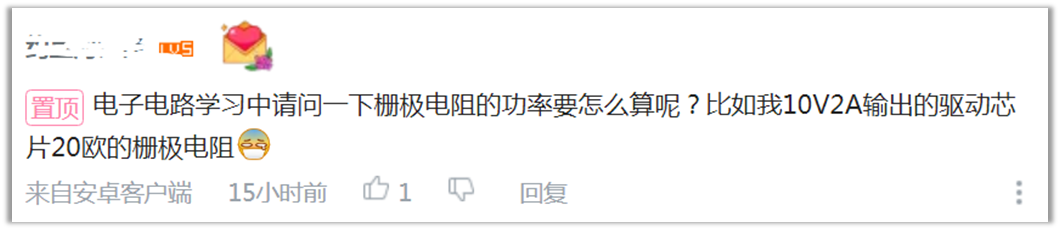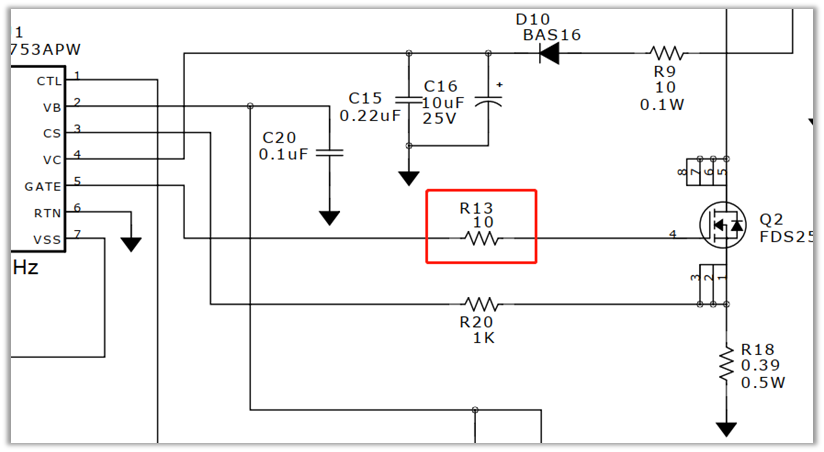B站是这个链接：https://www.bilibili.com/video/BV15o4y1o7Ru/

《LC串联谐振的意义》

MOS管串联电阻的问题，在视频的后面。两个链接内容是一样的，看一个就可以了。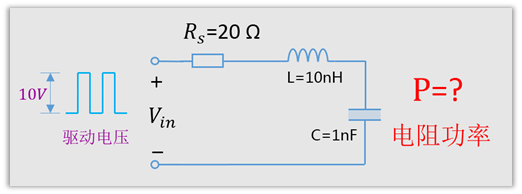What？这么复杂？你在玩我吗?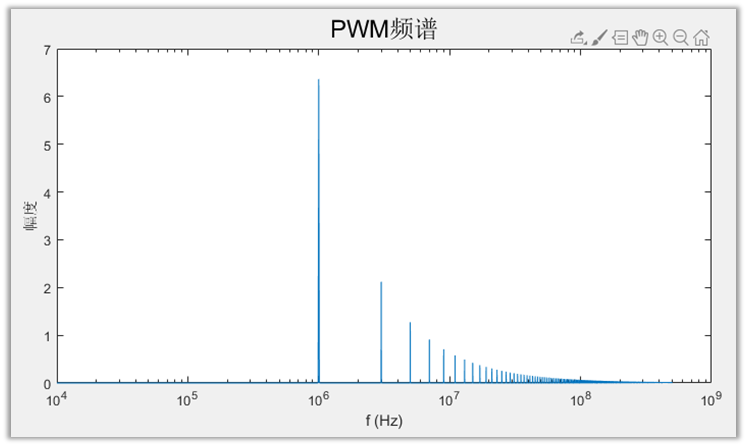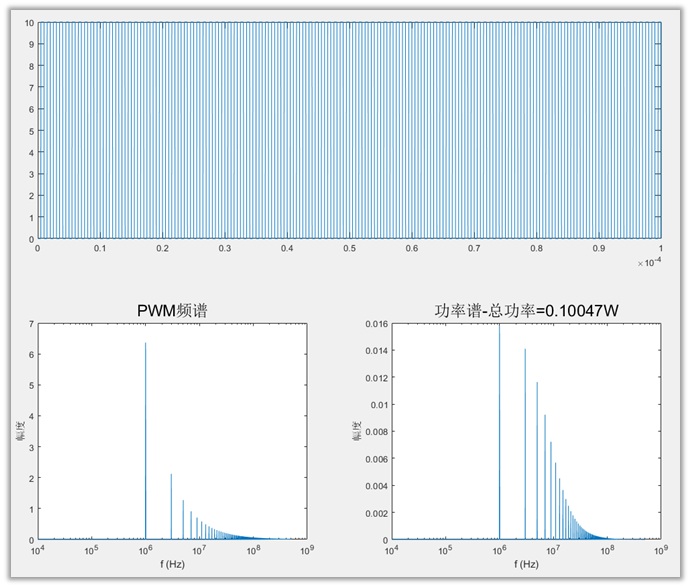Matlab代码

看不懂代码也没关系，这个模型最重要的参数就5个，电感，电容，电阻，驱动电压，开关频率。可以自己去尝试修改为不同的值，这样可以算出各种不同情况下电阻的功率。也可以收藏下，在以后真正需要计算的时候，代入相关参数，就可以得到电阻的功率啦。

``````Fclk=1000000; %PWM频率为1MHz
U0 = 10;     %电压幅值10V
Rs = 20;     %串联电阻为20Ω
ESL= 10^(-8);  %走线电感10nH
C= 10^(-9);  %走线电感为1nF

Fs=1000*Fclk;  %采样率为基频的1000倍
Num_T=100;     %分析100个周期的信号
L=(Fs/Fclk)*Num_T;   %100个周期信号长度(采样总点数)
T=1/Fs;    %采样周期
t=(1:L)*T; %时间长度

N=length(t);
LEN_PWM=zeros(1,N);  %定义PWM信号采样序列
for i=1:N            %产生PWM信号-点序列
if  mod(i,(Fs/Fclk))<Fs/(2*Fclk)
LEN_PWM(i)=0;
else
LEN_PWM(i)=U0;
end
end

figure;
subplot(2,2,[1 2]);
plot(t,LEN_PWM);      %画出PWM信号
title('PWM波形','FontSize',18);
set(gca,'XLim',[0 10/Fclk]);%x轴的数据显示范围
set(gca,'YLim',[-0.5 10.5]);%y轴的数据显示范围
xlabel('时间t (s)','FontSize',18);
ylabel('电压/V','FontSize',18);

X_LEN_PWM=abs(fft(LEN_PWM));   %fft傅里叶变换
Fn=Fs*(0:(L/2))/L;          %各个谐波频率序列
An=X_LEN_PWM(1:L/2+1)*2/L;  %每个谐波的幅值序列

Zn=Rs+Fn.*ESL.*2.*pi.*1i+1./(Fn.*C.*2.*pi.*1i);  %各个频率对应的总阻抗序列
Pn=(((0.707.*An)./abs(Zn)).^2)*Rs;  %各个频率的功率序列，电压有效值除以阻抗得到电流
Pall=sum(Pn)   %总功率

subplot(2,2,3);
semilogx(Fn,An);
title('PWM频谱','FontSize',18);
xlabel('f (Hz)','FontSize',18);
ylabel('幅度','FontSize',18);

subplot(2,2,4);
semilogx(Fn,Pn);
title(['电阻功率谱-总功率=',num2str(Pall),'W'],'FontSize',18);
xlabel('f (Hz)','FontSize',18);
ylabel('幅度','FontSize',18);``````

0/200